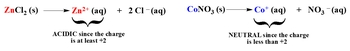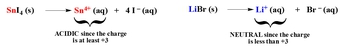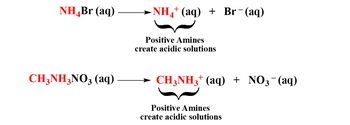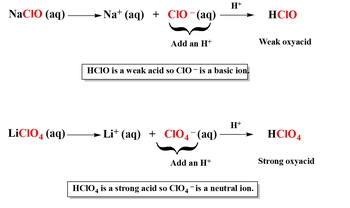## General Chemistry

Learn the toughest concepts covered in Chemistry with step-by-step video tutorials and practice problems by world-class tutors

17. Acid and Base Equilibrium

# Ionic Salts

When an acid and base undergo a neutralization reaction they form ions, which can create acidic, basic or neutral solutions.

Acid – Base Neutralization

Cations (positive ions) can create either acidic or neutral solutions.

1
concept

## Transition Metal Cations and Acidity3m
Play a video:
Hey, guys, In this brand new video, we're gonna take a look at salts. So what we should realize here is whenever we have an acid or base neutralizing one another, we're gonna usually form water as well as a second product. We call this second product assault, and let's think of a simple, basic type of acid based reaction. Let's think of hydrochloric acid, a very common strong acid, and it reacts with sodium hydroxide. N a O. H. Now remember our products you are going to be remember HCL is made up of h positive and cl negative. N a O. H is made up of any positive and O h minus. Opposites attract this positive in this negative are now attracted together. Whenever you have an H positive and O H minus, combining you form water. Now the N a positive and seal minus will be attracted to one another and they give us an A C l. We're gonna say n hcl is our salt. It's basically the ionic product that results from the neutralization between an acid and a base. Now we're going to say that this salt here, which is made up of any positive and seal minus. This salt could make our solution acidic, basic or neutral, depending on the rules that we're going to see in a few seconds from these two ions. That's the whole purpose of this section. So remember, we're gonna have to learn that salts are just ionic compounds. They're made up of a positive ion called a cat ion and a negative ion called an anti on. Now, based on certain properties, these ions could be acidic, basic or neutral. So let's take a look at it. We're gonna say here, if we're taking a look at our cat ions, we're gonna break down our cat ions into basically three categories. We have our transition metals. We have our main group metals, and then we have our positive amines. Remember, on your periodic table, the transition metals are the ones that are found within the pit. And remember, our main group metals are just group one A two a three a and four a medals, and then our positive amines, remember, Inamine is a compound that has nitrogen and hydrogen or carbon, hydrogen and nitrogen. So let's look over the first section. The rule says, if your transition. Metal has a charge off, plus two or higher. It's going to be acidic. We're gonna say if the charges less than plus two, then it's going to be neutral. So let's think of an example here. Let's say we have zinc chloride and then we have silver bromide. Now remember, zinc is always plus two. So when this thing breaks up into its ions, these are the ions were going toe create. Now zinc is a transition metal. It meets the requirement. It meets the minimum of a of A plus to charge. And because of that meets the minimum minimum of a plus to charge. It's going to be acidic now. Silver bromide. When it breaks up, it's gonna break up into a G plus. Plus B are negative now. Technically, if you remember, your side ability rules. Silver bromide is insoluble. Shouldn't break up. But we're just saying, theoretically, if it did break up here, it's a transition metal. It doesn't meet the requirement. It has to be plus two or higher. This is only plus one. So instead of being acidic, it would be neutral. All right, So for each of these rules that we're gonna go over. If they meet the requirements of them, they could be acidic or basic. If they don't meet that requirement, that means they're automatically going to be neutral.

If the transition metal within an ionic compound has a charge of +2 or higher then it creates an acidic solution. If it’s less than +2 then it creates a neutral solution and is classified as a non-significant ion.2
concept

## Main Group Metal Cations and Acidity1m
Play a video:
Now, if we go to the next one, we're gonna say if your main group metal has a charge of plus three or higher, then it's going to be acidic. If it doesn't meet that minimum requirement of plus three, it's going to be neutral. So if we have silver on the aluminum acetate, remember, we're only focused on the medals right now. We'll learn about what do we do with the negative ions in a few minutes. So we have aluminum acetate here, and then we have barium oxide. So remember this will break up into a little three positive on. We know what's three positive, because aluminum is in Group three A. And don't worry about a state. For now, we'll talk about how we look at that in a few minutes. So aluminum's a man group metal. It meets the minimum requirement of plus three. So it's going to be acidic. Barium oxide, barium and group to a. So it's plus two oxide is in Group six A. So it's minus two. This right here is the main group metal, but it doesn't meet the minimum requirement of plus three, and because of that, it will be neutral won't be acidic. So remember transition metals, the ones in the pit have to be plus two or higher, but main group metals have to be plus three or higher. If they meet the requirements, then they're acidic. If they don't meet those requirements, then they're going to be neutral.

If the main group metal within an ionic compound has a charge of +3 or higher then it creates an acidic solution. If it’s less than +3 then it creates a neutral solution and is classified as a non-significant ion.3
concept

## Positive Amines and Acidity4m
Play a video:
now positive amines are acidic. So let's think of some examples here we could have NH four br We could have ch three NH three cl. We could have C six h five NH three n 02 All different examples on what you should realize here is you just have to think of it like this. You could say that this first compound could be in a mean, but what's messing it up? That bro Ming is messing it up. That one element there signifies that we have assault, so you should know to break it up into its ions. So this breaks up into NH four. Positive. This breaks up into B are negative. We have a positive, I mean so therefore that, I mean, is automatically acidic. So remember, just tell yourself it could be in a mean. It could fit either this description or this description, but there's something that's interfering with it. What's that one thing? It's usually going to be at the end, and it's going to be a negative, an ion. So just break it up into its two ions. You'll have your positive of me and you'll have your negative ion. You're an eye on Look at the positive mean it's automatically going to be acidic. This to this could be a mean, but what's messing it up? The chlorine at the end? So it's assault. So break it up. We know it has to be plus one, because the halogen Zara Group seven A and there minus one. And look, we just isolated another positive. I mean, therefore, it's a cynic, just like this one is acidic. Finally, the last one, we could say that this portion here could be our mean, but what's messing it up? N O. To remember N 02 is our nitrite ion all those probably atomic on and you guys have to learn earlier on. You still have to remember them. So here, that's going to be C six h five NH three plus one. This is going to be N O to minus one again, let's not worry about the negative ions just yet. What's important for right now is that we have a positive I mean isolated, so it's going to be acidic. So remember, if we have transition metal, it needs to be plus two or higher to be acidic. If it's not. It's neutral for men, group medals, medals and groups. One a two a three a and four A. They have to be plus three or higher, or they're going to be neutral. And for the main group medals, if you really think about it, since the main group metals have to be plus through your or higher, that automatically means that group one A, which are always plus one and group to a, which are always plus two, will always be neutral. So they're not gonna meet the requirement. So if you see a assault question on your examine that has a group one A or to a metal involved automatically, that metal's going to be neutral because it's not gonna meet the minimum requirements. Group three X Plus three. So if you see aluminum or gallium or something, those will be acidic. It gets a little tricky and group for a. If you have a periodic table near you, just take a look at it in Group four A. We have 10 s n, and then we have led. Now they're in Group four A. But remember I said this several videos ago that these two, even though their main group, metals. They can act like transition metals that could be either plus two or plus four. So be careful. Which one are they giving you? If they're giving us M Plus two or P B Plus two, their main group metals, they don't meet the minimum requirement of plus three, so they would be neutral. But if you have sm plus four or P B plus four, then they wouldn't meet the minimum requirements and they would be acidic. So that was a little tricky. So remember that.

Amines are compounds with only nitrogen or hydrogen (i,e. NH3) or with carbon, nitrogen and hydrogen (i,e. CH3NH2). Positive amines within compounds create acidic solutions.Anions (negative ions) can create either basic or neutral solutions.

4
concept

## Anions and Basicity3m
Play a video:

To determine if an anion is basic or neutral you must add an H+ to it. If adding an H+ creates a weak acid then your negative ion is basic, but if adding an H+ creates a strong acid then your negative ion is neutral.5
example

## Ionic Salts (Simplified) Example 12m
Play a video:
Hey, guys, in this new video, we're gonna put to practice some of the concepts we learned about salts when it comes toe acid base neutralization. So let's take a look at this first example we're gonna say here, determine if each of the following compounds will create an acidic, basic or neutral solution, so we're gonna have to break them up into their ions. We're gonna take a look at the positive ion and see if it's acidic or neutral, and they're gonna take a look at the negative ion and see if it's basic or neutral. So for the first one, we're gonna say it's n a o C E o. Now, remember, this is easy to break up. The metal always breaks off by itself, and we know that a is plus one because it's in Group One A. And then our negative ion would be OSI all negative. Remember, if we take a look at the positive ion en is a main group metal main group metals have to be plus three or higher to be acidic because this is only plus one, it fails to meet the requirement, so it's neutral. Now let's take a look at the negative ion. Now for this negative ion, we're gonna have to add an H plus to it. So we get Hochul every time you add in h two it, you're gonna create an acid. This one has an oxygen in it. So we just created an oxy acid. Do the math. If you do one oxygen minus one Hydrogen, you have nothing left. So this would be a week. Oxy acid, Remember? What does the rules say? It says if you create a weak acid, then your negative ion will be basic. And since we just created a week oxy acid, that means that this negative ion is basic. So we have a neutral ion. We have a basic ion neutral means we can ignore it. That means it doesn't affect the solution at all. So we're gonna look at the basic ion. The basic ion will make our solution basic. It's a simple as that. So if you have a neutral on the basic, it gives you basic. If you have a neutral and acidic, it gives you acidic. If you have neutral and neutral, you're gonna be neutral Overall And then in some rare cases, we can have. Ah, Citic and basic. We'll take a look on. How exactly do we approach that later on down this page?
6
example

## Ionic Salts (Simplified) Example 21m
Play a video:
So for the next one, we have P B C. L four. So we have to break this up into its ions. Okay? So just realize here you see this little four that came from the lead. So it's led four positive plus chloride ion, which is CR minus. Are you going to say now is this is a main group metal because it's in Group four A. And it's plus four man group metals have to be plus three or higher to be acidic. This one is definitely within the requirement. So it's gonna be acidic. This c O minus at NH positive to it. You create an acid since it doesn't have any oxygen's, it's a binary acid. And remember, HCL is one of the three strong binary acids, and we're gonna say here if you create a strong acid thin, the negative ion has to be neutral because it goes against the rule that we talked about so this year would be neutral so we can ignore it. So we said earlier, if you have an acidic and a neutral, you're acidic overall, so this is the approach you have to take with each of these, so I want you to take a look at practice one for this one. You need to do the same thing. You need to break those two compounds into their ionic forms, then use the rules that we've gone over. Once you do that, you can figure out if they're acidic, basic or neutral. Good luck on this. First one, guys.
7
Problem

Determine if each of the following compounds will create an acidic, basic or neutral solution.

a) LiC2H3O2   b) C6H5NH3Br

8
Problem

Determine if each of the following compounds will create an acidic, basic or neutral solution.

a) Co(HSO4)2           b) Sr(HSO3)2

9
Problem

Determine if each of the following compounds will create an acidic, basic or neutral solution.

a. C3H7NH3F

10
example

## Determine whether each compound will become more soluble in an acidic solution3m
Play a video:
Hey, guys, in this brand new video, we're gonna continue with our discussion off salts. And how exactly do they relate toe finding pH or the P O h of a solution? So for this first example, it says determine whether each compound will become more soluble in acidic solution. Now, this question is different from anything we've seen thus far. But remember, the key to this is the one that has a basic ion. So basically a basic negative ion will beam or soluble in acidic solution. This has to do with le chateau leers principle. So if we create a basic eye on and we throw it into a civic solution, the acids going to destroy it. So what we have to do is the product at the reaction has to continuously make mawr of the basic ion to keep up with it being destroyed. This increases the solid ability of the whole compound. So, for this question is, we're gonna say it's more Sybil in acidic solution when you have a basic ion. So we're looking for the one that has a basic ion this first one. If we break it up, it breaks up into in a positive b r negative. I remember The positive ions are either acidic or neutral, so we know we're not looking at them. It's the negative ions that could be basic. And if they're not basic, then they're neutral. Here we have BR minus. We've seen this before, just at an H plus to it, we get HBR. That's a strong binary acid, so this would be neutral, so this would not become more soluble in acidic solution. Here this breaks up into L. A positive plus CR minus. Again, it's a C l minus. So add an H plus to it. We get hcl again. We have a strong binary acid because we have a strong binary acid. This negative ion would be neutral. Also remember, we're looking for the basic ion and only negative ions can be basic. This breaks up into K positive, plus I o negative at an H plus to it. We create a church, i o. Now it has an oxygen in it. So it's an oxy acid. So we have to do is do the math. We have one oxygen minus one hydrogen. So we have nothing left. So that would be a week oxy acid. And because it's a week oxy acid. This ion here is basic. We're gonna say basic ions are going to react with the acidic solution decreasing the amount of this taking it out. So what has to happen are reacting here. Has to continuously break up to replenish what it just lost so as to remake the i o negative. So if it's continuously breaking down to make more of it, it's soluble. Sybil means that the compound your reacted is breaking down or dissolving in your solution. So see would have to be the answer. Okay. And the same thing could work for a basic solution. You're gonna say you're more Sybil in a basic solution. If you have a positive ion, that is acidic, so just remember that
11
example

## Determine the pH of a 0.50 M NH4Cl solution7m
Play a video: